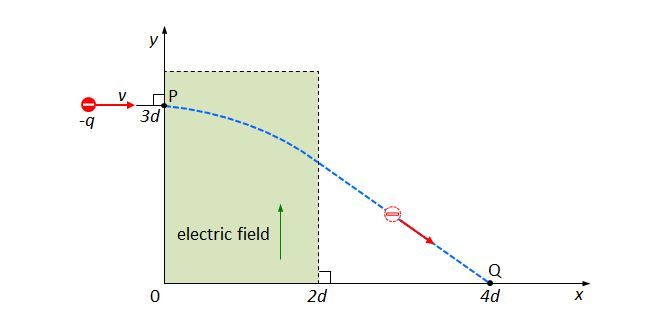# Moving charged particle 2A particle with electric charge $-q$ enters a uniform electric field at the point $P=(0, 3d).$ The direction of the electric field is the $+y$ direction. The charged particle moves along a projectile path inside the electric field. After exiting the electric field, it shows a uniform motion, arriving at $Q=(4d, 0).$ If another charged particle with the same mass but a different electric charge of $-2q$ enters the electric field in the same way as above, what will be the destination point on the $x$-axis?

Ignore the gravitational force and the sizes of the charged particles.

×